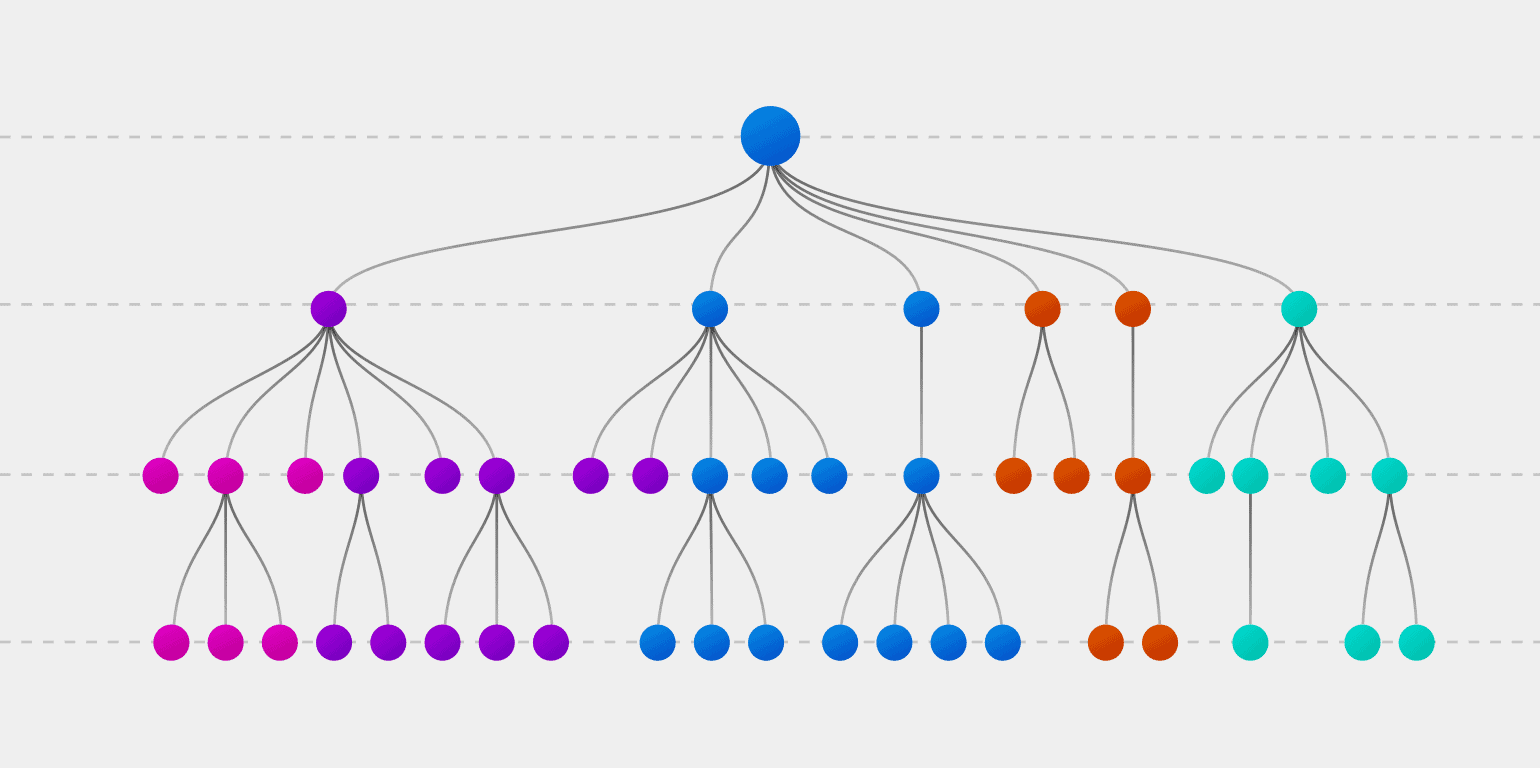Decision Tree is one of the beautiful algorithms used for solving both classification and regression problems. It is a supervised machine learning algorithm, which means along with the independent features, output features/labels are required for building the decision tree-based model.

A decision tree is a powerful algorithm and used for solving complex datasets. It became popular due to its simplicity, as it is easily understandable and has a good tree-like visualization of the entire model. Decision Tree forms the base of most ensemble techniques like Random Forest, Adaboost, Xgboost.Decision Tree

Unlike linear regression, where it tries to find the relationship between independent and dependent features, a Decision tree follows an approach of dividing the dataset based on rules, thereby constructing trees up to their complete depth.

Let us consider an example of a health tracking app, based on certain parameters it tries to give output whether a person needs a health check-up or not. Heartbeat rate, pulse rate, sleeping duration, water intake, food calories are the independent features and Need checkup(Yes/No) is a dependent feature. The decision tree tries to build a tree-like structure, for which some independent features should be parent node (root node), some needs to child node and some needs to be leaf node where decisions are taken. Now it is important to find out, which one of the independent features is to be chosen as a parent node, either it can be heartrate or pulse-rate or sleeping duration or water intake, food calories. For this decision, Entropy, information gain or Gini impurity comes into the picture.

Entropy and Information Gain:

Entropy is a measure of randomness in the dataset. It also calculates the impurity in the dataset. Say for eg. atoms in solids are structured & randomness is less, which means in solids entropy is low. In the case of gases, atoms are sparse and randomness is more, which shows entropy is more for gases.

Entropy is calculated for all the independent features. This entropy is further used for the calculation of information gain. With the help of information gain, the parent node and subsequent child node will be decided.

Information Gain:

Information gain for each independent feature is calculated using the above equation. After calculation of information gain for every independent feature, the feature which has more information gain forms the parent node and other features will be child node. The child node is further split until it reaches the leaf node.

Ginni impurity:

Ginni impurity is calculated by multiplying the probability of given observation is classified into correct class and sum of all probability of that particular observation classified into the wrong class.

Ginni values lie between 0 and 1. 0 being no impurity and 1 being randomly distributed. The feature with a low Ginni value is chosen as a parent node to split.

Different algorithms for Decision tree:

Different algorithms used for building decision tree includes ID3 (uses ginni & for classification problem and takes only categorical attributes), C4.5 (uses ginni & for classification problem and takes both continuous, discrete and categorical attributes), CART(Classification and Regression Tree) (uses ginni, also option to change to entropy, solves both classification and regression problems), C5, MARS, Decision Stump, M5, Conditional inference tree (CIT), CHAID.

Tree Pruning:

Tree pruning is a method to reduce the complexity of the tree or reduce the high variance in the model. Pruning is similar to regularisation in Linear regression, where Ridge, Lasso and Elastic net are used for avoiding overfitting of the model. Tree pruning can be either pre-pruning(forward) or post-pruning(backward).

Pre-pruning, the non-significant branches are stopped before tree generation. In case of post-pruning, the tree is allowed to grow first, then non-significant branches are removed using Gridsearch cv which selects optimal hyperparameter.